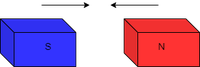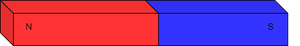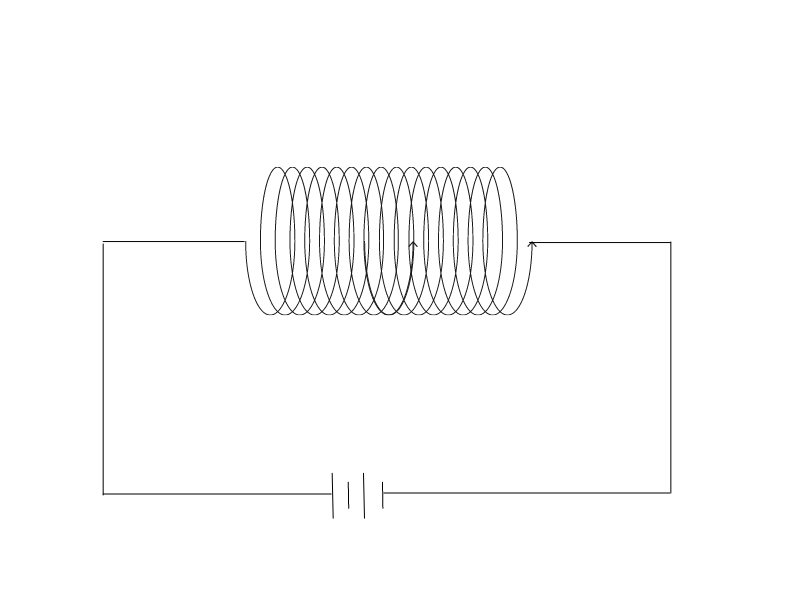# Bar Magnet as an Equivalent Solenoid

• Last Updated : 15 Mar, 2022

A permanent magnet is a bar magnet, but an electromagnet is a solenoid that operates as a magnet only when an electric current is supplied through it. When a bar magnet is split in half, both parts behave like magnets with the same magnetic characteristics, however, when a solenoid is sliced in half, the magnetic fields are weaker.

The poles of a bar magnet are fixed, but the poles of a solenoid may be changed. The strength of a bar magnet’s magnetic field remains constant, but the strength of a solenoid’s magnetic field is dependent on the electric current that passes through it.

### What is Magnetism?

Magnetism is the property of a matter to get attracted or repelled from other matter. This property depends on the movement of electric charges.

Actually, electricity and magnetism are interrelated to each other. That’s why the branch of physics which studies the electric and magnetic fields together is known as electromagnetism. Every substance has a number of atoms inside and many numbers of electrons. But initially, it is electrically neutral. When it gets charged it generates an electric field and corresponding magnetic field.

Types of magnetism present are:

• Ferromagnetism
• Ferrimagnetism
• Anti-ferromagnetism
• Para magnetism
• Diamagnetism
• Super-para magnetism

### Magnet

Magnet is a material present in this world that gets attracted or repelled by other objects and it produces a magnetic field around it. There are two poles in magnet: North pole and South pole

• N – N = repels
• N – S = attracts
• S – N = attracts
• S – S = repelsAttraction of magnets

Magnets are always present in bipolar form and these poles can not be isolated. If we try to isolate these poles by breaking the magnet into pieces then it will not be isolated. In fact, now each and every piece of the magnet will have its own individual north pole and south pole.

There are basically two types of magnets:

1. Permanent magnet: These magnets have an internal structure such that they attain magnetism permanently which means the magnetic field is always present. e.g. Bar magnet
2. Temporary magnet: These magnets only attains magnetism temporarily whenever they are in contact with some permanent magnet or electricity. e.g. electromagnet

As matter can be present in any state so magnet can also be in different state. e.g.

• Solid-State: Neodymium Magnet,
• Liquid State: Ferrofluids, and
• Gaseous state: Actually strong magnetic property is not seen in a gaseous state. In gaseous state paramagnetic material can be found not a ferromagnetic material. An example of paramagnetic gas is Oxygen.

### Bar Magnet

A bar magnet is a cuboidal-shaped object which is made up of certain ferromagnetic materials. It has two poles in it one is the north pole and another is the south pole.

Most of the time half part of the magnet which is the north pole is colored with red color and other parts of the magnet are colored with blue color which behaves as the south pole.

Materials used in making Bar magnets are iron, nickel, cobalt, or any other ferromagnetic materials. There are two poles in Bar magnet: The North pole and the South pole.

• N – N = repels
• N – S = attracts
• S – N = attracts
• S – S = repelsSimple bar magnet

Formula used for Bar Magnet-

Pole strength of the magnet is given by,

P = W / I

where

• W is work done by the magnet to move around the wire, and
• I is the current flowing through the wire.

The S.I. unit is N.Tesla-1.

Example 1: If the work done by the magnet to move around the wire is 30 J and the current flowing through the wire is 15 A. Find the pole strength.

Solution:

We know that formula of pole strength is,

P = W/I

= 30 / 15

= 2 N.Tesla-1

Properties of Bar Magnet

• Bar magnet produces a magnetic field, the magnetic field lines are from one pole to another pole of the same magnet.
• When suspended freely, the north of the magnet is directed towards the north pole of earth where magnetic south of earth is present and the south of the magnet is directed towards the south pole of the earth where magnetic north is present.
• The density of magnetic field lines is maximum at poles so magnetism is stronger at poles.
• The magnetic field lines produced by bar magnets do not intersect.

Uses of Bar Magnet

• Large Bar magnets can be used in garbage sites for doing magnetic separation of garbage.
• Bar magnets have scope in the medical field in curing diseases.
• Bar magnets are used in laboratories for various purposes.
• Bar magnets can be used in making compasses for finding correct directions.

### Solenoid

The solenoid is a device that is made using a wire which is twisted around to make a coil of a certain number of rounds.

When an electric current is flown through a solenoid it acts as a magnet and generates a magnetic field around it and acquires magnetic property but as soon as electricity stops it loses its magnetic property. So, it is an example of a temporary magnet.Solenoid

#### Formula for Magnetic field of a Solenoid-

The magnetic field of a solenoid is given by,

B = μo NI / l

where

• μo is magnetic constant equal to 4π × 10-7
• N is the number of rings in coil
• I is the current and
• l is the length of the solenoid

The S.I. unit of magnetic fields is Tesla.

Example 2: Find the Magnetic field of solenoid if number of turns in coil is 200 current flowing is 1000 A and length of solenoid is 3.14 m.

Solution:

We know that formula for finding magnetic field is B =  μo NI / l

= 4π × 10-7 × 200 × 1000 / 3.14

= 4 × 10-7 × 2 × 105

= 8 × 10-2 T

Properties of a Solenoid:

• When electric current flows from ‘+’ terminal to ‘-‘ terminal. Solenoid starts behaving as a magnet and starts attracting nearby magnetic devices.
• The density of magnetic field lines is maximum at the ends of the solenoid so magnetism is stronger near the ends.
• The magnetic field lines produced by solenoids do not cut each other.

Uses of a Solenoid:

• It is used in computer hard disk drives to store memory
• It is used in making an electric bells.
• It is used in making valves used in Air conditioning system
• It is used in making MRI machines.

### Sample Questions

Question 1: Define magnet and magnetism.

Magnet is a material present in this world which gets attracted or repelled by other objects and it produces a magnetic field around it. There are two poles in magnet : North pole and South pole.

Magnetism is property of a matter to get attracted or repelled from other matter. This property depends on movement of electric charges.

Question 2: If a magnet with a pole strength of 5 is bought near a current carrying conductor with current 20 A. Find the work done in doing so.

We know that, P = W/I

W = P.I

= 20 × 5

= 100

So, the work done in moving magnet is 100 J.

Question 3: State behaviour of two bar magnets at four different positions.

Two bar magnets can be placed in four different positions:

• North facing North
• North facing South
• South facing North
• South facing South

Their behaviour are as follows:

1. N – N = repels
2. N – S = attracts
3. S – N = attracts
4. S – S = repels

Question 4: Find the magnetic flux of a disk of area 20 m2 having 5 as magnetic field. Given angle between area vector of disk and direction of magnetic field is 60o.

The formulae for magnetic field is , φ = B A cosθ

= 5 × 20 × cos60°

= 5 × 20 × 1/2

= 50 Maxwell

So, the magnetic flux of given disk is 50 Maxwell.

Question 5: Write uses of bar magnet.